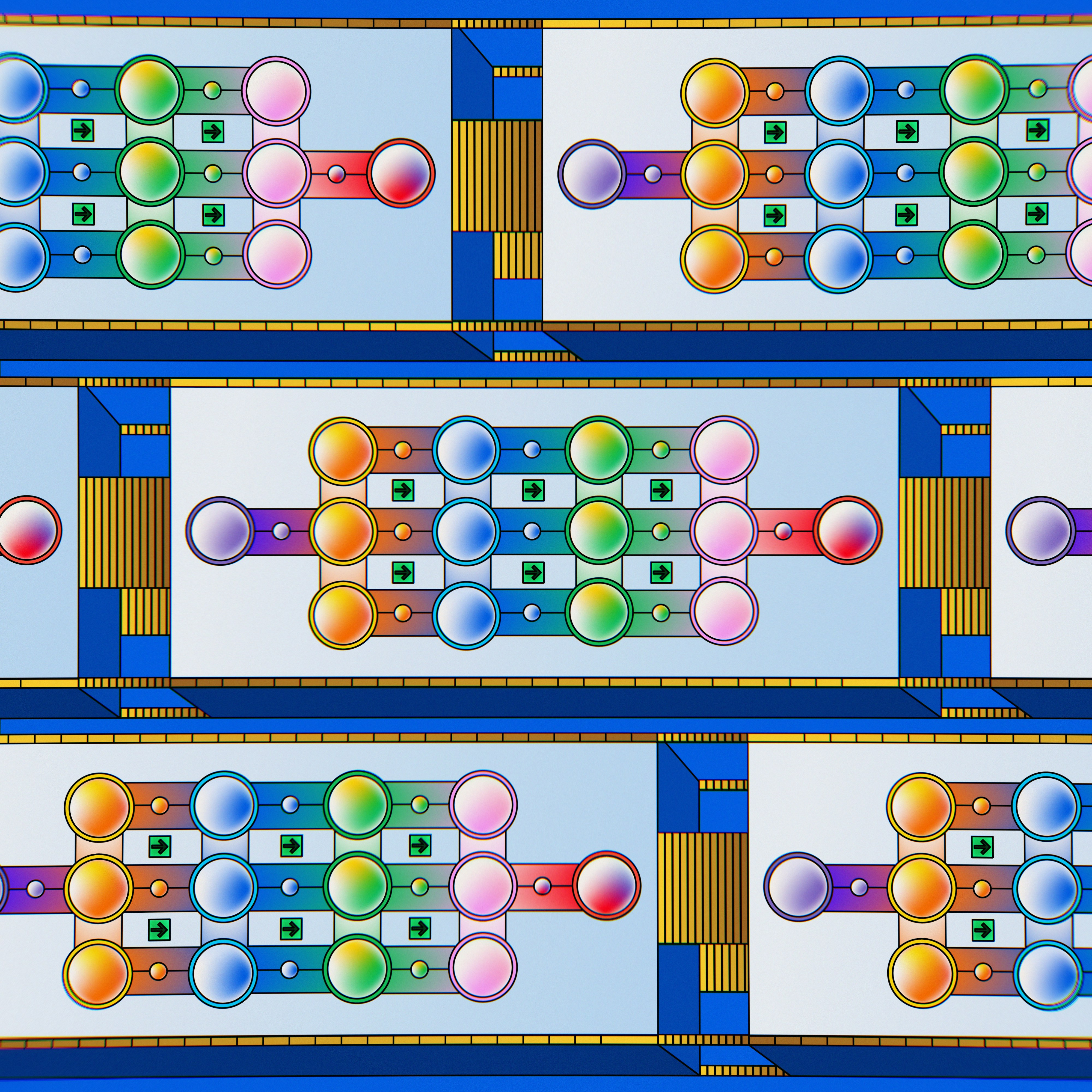## 机器学习：模型动态增量训练

2983 字 · 51 阅读 · 2023 年 05 月 20 日• 动态模型
• 增量训练
• 实时手写字符识别

### 静态模型和动态模型

• 静态模型采用离线训练方式。一般只训练模型一次，然后长时间使用该模型。
• 动态模型采用在线训练方式。数据会不断进入系统，通过不断地更新系统将这些数据整合到模型中。scikit-learn 中， 的算法有：

• 分类算法
• sklearn.naive_bayes.MultinomialNB
• sklearn.naive_bayes.BernoulliNB
• sklearn.linear_model.Perceptron
• sklearn.linear_model.SGDClassifier
• sklearn.linear_model.PassiveAggressiveClassifier
• sklearn.neural_network.MLPClassifier
• 回归算法
• sklearn.linear_model.SGDRegressor
• sklearn.linear_model.PassiveAggressiveRegressor
• sklearn.neural_network.MLPRegressor

from sklearn.datasets import load_digits

digits.data.shape, digits.target.shape


from sklearn.model_selection import train_test_split

X_train, X_test, y_train, y_test = train_test_split(
digits.data, digits.target, random_state=1, test_size=0.2)

X_train.shape, X_test.shape, y_train.shape, y_test.shape


from sklearn.metrics import accuracy_score
from sklearn.neural_network import MLPClassifier

model = MLPClassifier(random_state=1, verbose=1, max_iter=50)

model.fit(X_train, y_train)  # 训练模型
y_pred = model.predict(X_test)  # 测试模型
accuracy_score(y_test, y_pred)  # 准确度


n = 0
for i, (pred, test) in enumerate(zip(y_pred, y_test)):
if pred != test:
print('样本索引:', i, '被错误预测为: ', pred, '正确标签为: ', test)
n += 1
print("总计错误预测样本数量:", n)


from matplotlib import pyplot as plt
%matplotlib inline

plt.imshow(X_test.reshape((8, 8)), cmap=plt.cm.gray_r)


### 动态增量训练

scikit-learn 中，增量训练的方法是 model.partial_fit(X, y)，其使用方法与 model.fit(X, y) 别无二致。

import numpy as np

for i, (pred, test) in enumerate(zip(y_pred, y_test)):
if pred != test:

# 增量训练模型
model


y_pred = model.predict(X_test)  # 测试模型
accuracy_score(y_test, y_pred)  # 准确度

# 打印错误预测样本
n = 0
for i, (pred, test) in enumerate(zip(y_pred, y_test)):
if pred != test:
print('样本索引:', i, '被错误预测为: ', pred, '正确标签为: ', test)
n += 1
print("总计错误预测样本数量:", n)


from IPython.display import HTML

input_form = """
<table>
<td style="border-style: none;">
<div style="border: solid 2px #666; width: 43px; height: 44px;">
<canvas width="40" height="40"></canvas>
</div></td>
<td style="border-style: none;">
<button onclick="clear_value()">重绘</button>
</td>
</table>
"""

javascript = '''
<script type="text/Javascript">
var pixels = [];
for (var i = 0; i < 8*8; i++) pixels[i] = 0;
var click = 0;

var canvas = document.querySelector("canvas");
if (e.buttons == 1) {
click = 1;
canvas.getContext("2d").fillStyle = "rgb(0,0,0)";
canvas.getContext("2d").fillRect(e.offsetX, e.offsetY, 5, 5);
x = Math.floor(e.offsetY * 0.2);
y = Math.floor(e.offsetX * 0.2) + 1;
for (var dy = 0; dy < 1; dy++){
for (var dx = 0; dx < 1; dx++){
if ((x + dx < 8) && (y + dy < 8)){
pixels[(y+dy)+(x+dx)*8] = 1;
}
}
}
} else {
if (click == 1) set_value();
click = 0;
}
});

function set_value(){
var result = ""
for (var i = 0; i < 8*8; i++) result += pixels[i] + ","
var kernel = IPython.notebook.kernel;
kernel.execute("image = [" + result + "]");
kernel.execute("f = open('digits.json', 'w')");
kernel.execute("f.write('{\\"inputs\\":%s}' % image)");
kernel.execute("f.close()");
}

function clear_value(){
canvas.getContext("2d").fillStyle = "rgb(255,255,255)";
canvas.getContext("2d").fillRect(0, 0, 40, 40);
for (var i = 0; i < 8*8; i++) pixels[i] = 0;
}
</script>
'''
randint = np.random.randint(0, 9)
print(f"请在下方图框中细心绘制手写字符 {randint}")
HTML(input_form + javascript)


import json
import numpy as np

with open("digits.json") as f:
plt.imshow(inputs_array.reshape((8, 8)), cmap=plt.cm.gray_r)


# 重新训练神经网络
digits.data[digits.data > 0] = 1
model = MLPClassifier(tol=0.001, max_iter=50, verbose=1)
model.fit(digits.data, digits.target)


inputs_array = np.atleast_2d(inputs_array)  # 将其处理成 2 维数组
result = model.predict(inputs_array)  # 预测

if result != randint:
print(f"预测错误｜预测标签: {result}｜真实标签: {randint}")
model.partial_fit(inputs_array, np.atleast_1d(randint))
print("完成增量训练")
else:
print(f"预测正确｜预测标签: {result}｜真实标签: {randint}")
model.partial_fit(inputs_array, np.atleast_1d(randint))
print("完成增量训练")


# 输出神经网络对各类别的概率值
pred_proba = model.predict_proba(np.atleast_2d(inputs_array))

# 绘制柱形图
plt.xticks(range(10))
plt.bar(range(10), pred_proba, align='center')


### 系列文章•